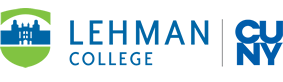Give

# Lehman College## Learning Goals and Objective

### B.A. in Mathematics

Upon completion of a B.A. in Mathematics, a graduate will be able to do the following:

• Perform numeric and symbolic computations
• Construct and apply symbolic and graphical representations of functions
• Model real-life problems mathematically
• Use technology appropriately to analyze mathematical problems
• State and apply mathematical definitions and theorems
• Prove fundamental theorems
• Construct and present a rigorous mathematical argument

To achieve these goals, courses required for the major are targeted for specific areas as outlined in the Lehman Math Majors Goals Site. Final exams in relevant courses will include problems directly testing this knowledge.